# How to Calculate Square root and cube root in Microsoft Excel

In this article, we will find out how to calculate the square root and cube root of a number. We will also find out the nth root of a number by using the POWER function in Excel 2016.

x, to the power of y, is referred to as xy.

The Root of a number is inverse of Power of the number.
Square of a number = Power(2) of a number
The square root of a number = power(½) of a number

 =>y=x2  =>(y)1/2 =x

SQRT function is used when we need to find the square root of a number in Excel.

Syntax of SQRT:

 =SQRT(number or cell reference)

POWER function is used when we need to find out the exponent of a number in Excel.

Syntax of POWER:

 =POWER(number or cell reference, 1/3)

Let’s take some examples as shown below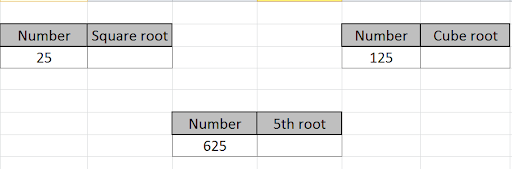Use the formula to find the square root of the number in cell B3.

 =SQRT(B3)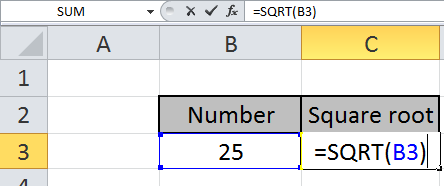Press Enter to see the square root of 25.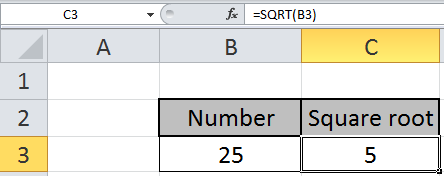The square root of 25 is 5.

To find out the cube root of a number, we will use the POWER function.
Write the formula in the desired cell.

 =POWER(F3,1/3)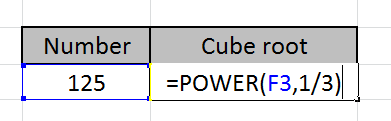Press keyboard key Enter to see the cube root of the number in cell F3.
Cube root of 125 is 5.

Let’s see below to find out the nth root of a number by using the above method.
Use the formula

 =POWER(D7,1/4)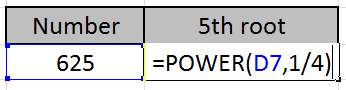Press key Enter to view your output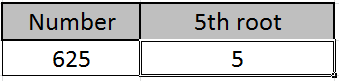Power(4) of 5 is 625.

So, 4th root of 625 is 5.

You can customize your formula with these basic functions. As you can see by using the mathematical function in Excel you can perform many exponents function. Please do find more articles on the mathematical formulation here.

Related Articles:

How to use the SQRT Function in Excel

Popular Articles:

50 Excel Shortcut to Increase Your Productivity

How to use the VLOOKUP Function in Excel

How to use the COUNTIF function in Excel 2016

How to use the SUMIF Function in Excel

## Users are saying about us...

1.i try but i just want to write the power on top just like m raise power 3 example m3 this 3 i want to write on the top of m thanks any one reply me thanks

2.This is how you get presented on papers usually.. some universally agreed format of math people to understand each other. In digital world you need to present figures for apps to understand. And this is how you give commands to them.. in their language agreed by programmers. Essentials of programming would help you more, I guess )

3.I use the formulat Log10 (4) to calculate the log base 10 of 4. How can I
compute the inverse of log 10.

4.In general, if y = log10(x), then x = 10^y.

So, if you have =LOG10(4) in cell A1, showing approximately 0.60206, then
enter =10^A1 in some other cell, which will show 4.

5.6.The cube of 5 is 125 not 225 / cube root of 125 is 5. Cube root of 225 is 6.08. The typo needs correcting, other than that it was helpful

•thank you, i always assume i'm wrong and this had my head spinning until i saw your confermation

Terms and Conditions of use

The applications/code on this site are distributed as is and without warranties or liability. In no event shall the owner of the copyrights, or the authors of the applications/code be liable for any loss of profit, any problems or any damage resulting from the use or evaluation of the applications/code.